# Modulus of an automorphism

(diff) ← Older revision | Latest revision (diff) | Newer revision → (diff)

A positive real number associated to an automorphism of a locally compact group. Letbe such a group and letbe an automorphism of, regarded as a topological group. Then the modulus ofis defined by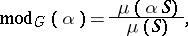whereis left-invariant Haar measure onandis any compact subset ofwith positive measure (indeed,does not depend on). Ifis compact or discrete, then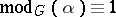, since for a compact group one can put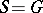, and for a discrete group one can take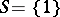, whereis the identity element of.

Ifandare two automorphism of, then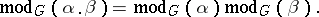Ifis a topological group which acts continuously onby automorphisms, then the associated homomorphism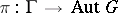defines a continuous homomorphism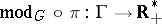, where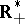is the multiplicative group of positive real numbers. In particular, if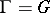and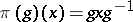, then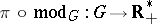is a continuous homomorphism. This homomorphism is trivial if and only if the left-invariant Haar measure onis simultaneously right invariant. Groups satisfying the latter condition are called unimodular.

Ifis a locally compact skew-field, then each non-zero element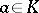defines an automorphismof the additive group ofvia multiplication by. The function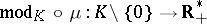is used in the study of the structure of locally compact skew-fields.

How to Cite This Entry:
Modulus of an automorphism. Encyclopedia of Mathematics. URL: http://encyclopediaofmath.org/index.php?title=Modulus_of_an_automorphism&oldid=15257
This article was adapted from an original article by L.V. Kuz'min (originator), which appeared in Encyclopedia of Mathematics - ISBN 1402006098. See original article